Introduction
Matrix Action
Perpframes, Aligners and Hangers
Stretchers
Coordinates
Projections
SVD
Matrix Subspaces
Linear Systems, Pseudo-Inverse
Condition Number
Matrix Norm, Rank One
Data Compression
Noise Filtering
###### Todd Will UW-La Crosse
Pseudo-inverse Example
Reduced SVD

Pseudo-inverse

# Pseudo-Inverse

Inverse and Determinant

Exercises

## Pseudo-Inverse Example

Suppose the SVD for a matrix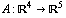is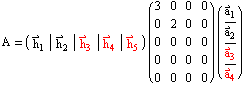.

How can you use the decomposition to solve the matrix equation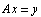Answer:  The first thing you know is that no matter what x you use, A x is always in the column space of A,  Col[A].

So if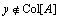, the equation won't have any solution.

Ifyou should solve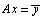where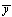is the vector in Col[A] closest to y

The solution tois called the least squares solution to A x = y

Here's what you do to solve the system:

Step 1: Find the vectorin Col[A] closest to y.

Since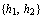is an orthonormal basis for Col[A], it's easy to compute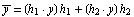(Note that if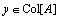, then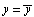.)

Step 2: Let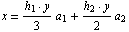That's it!

If the system has a solution, then you've just found it.

If the system has no solution, then you've done the best you can -- you've found the least squares solution.

Here's a check on your solution:

If you set,  then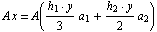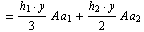(Linearity)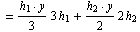(Fundamental Equations)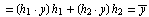Note that you can computeusing the matrix multiplication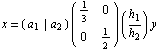The matrix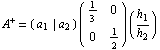is called the pseudo-inverse of A.

The least squares solution to A x=y is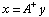Other least squares solutions have the form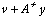where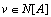Since the SVD gives you an orthonormal basis for N[A], you know any least squares solutions to A x=y can be expressed as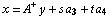## Reduced SVD

It's easiest to describe the pseudo-inverse in general terms by first defining the reduced SVD for A

Ifis an SVD of A

then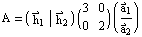is the reduced SVD for A

You get the reduced SVD from the full SVD by keeping only

• the non-zero singular values in the stretcher matrix

•
• the columns of the hanger and rows of the aligner corresponding to non-zero singular values.

You can tell that the reduced SVD equals the full SVD (and so still equals A), since the two decomposition agree on the basis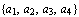## General Pseudo-Inverse

If you have the reduced SVD for an m x n matrix A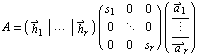then the pseudo-inverse of A is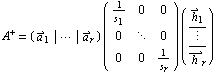.

The least squares solution to A x = y is given by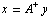## The Inverse and the Absolute Value of the Determinant

Suppose A is a square matrix and an SVD is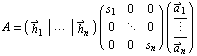.

As it turns out the absolute value of the determinant of A,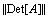equals the product of the singular values.

Thus, if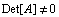, then you know that all of the singular values are positive.

This tells you that A has rank n and so is invertible.

If all the singular values are positive, then you can compute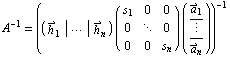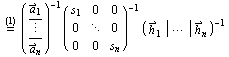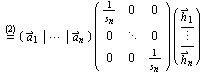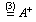(1) The inverse of a product is the product of the inverses in reverse order.

(2) The aligner matrix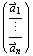takes the perpframe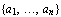to the standard basis.
The inverse of this procedure, taking the standard basis to the perpframeis accomplished by the hanger matrix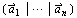A similar argument explains why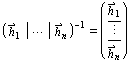To undo the stretcher matrix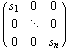stretches the standard basis vectors by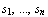You can reverse this by stretching the standard basis vectors by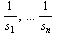This is done with the stretcher matrix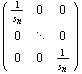which explains why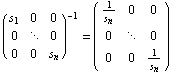.

(3) The definition of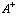Comment: You've just shown that if A is invertible, then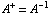## Exercises

1. Suppose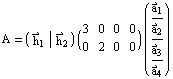is a full SVD for A.
In terms of the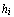and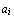a. express the reduced SVD for A as a product of three matrices

b. express the pseudo-inverse,as a product of three matrices

c. express a solution to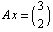d. express all solutions to2.  Supposeis a full SVD for A.

a. What are the possible values for the determinant of A?

b. In terms of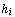andexpress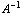as the product of three matrices.